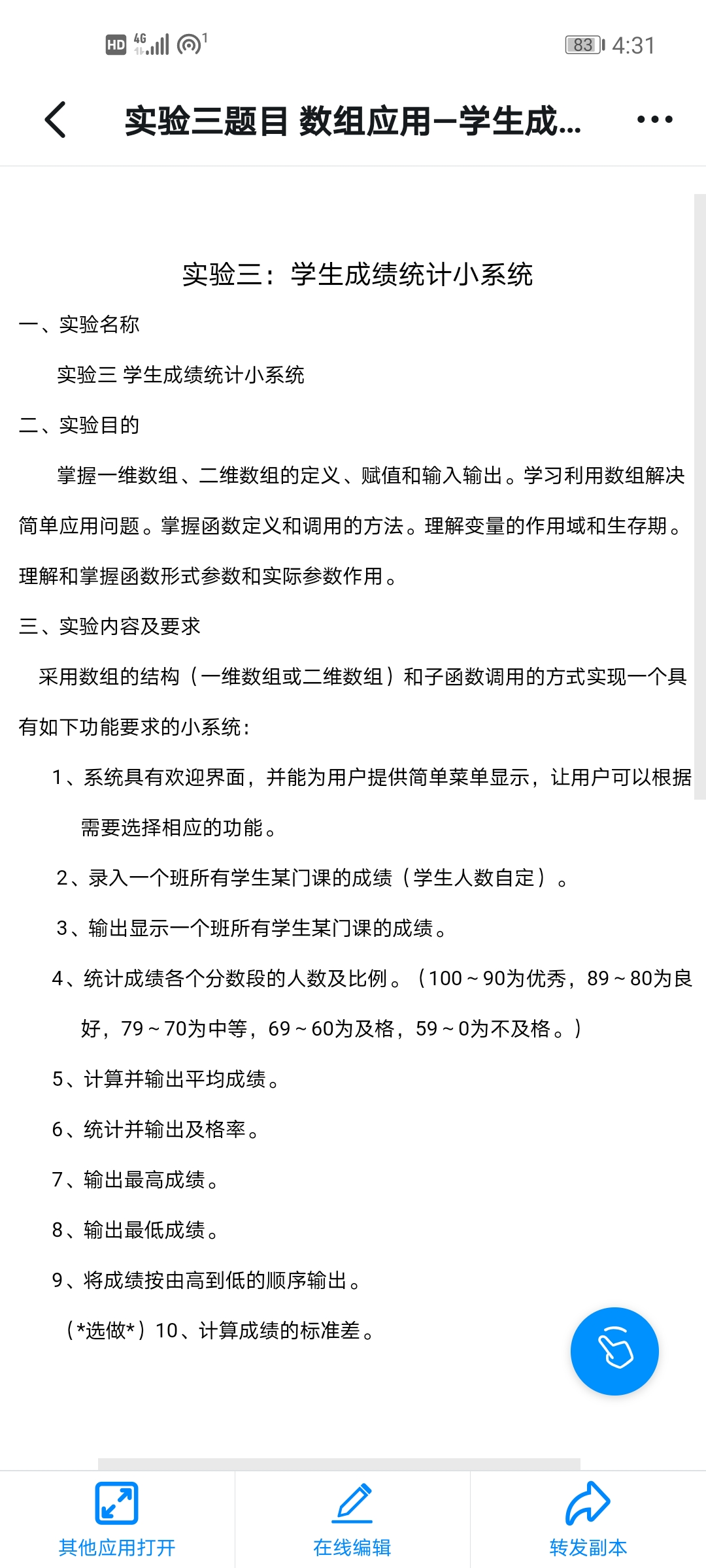2021-06-08 16:34

# c语言学生成绩统计系统• 点赞
• 写回答
• 关注问题
• 收藏
• 邀请回答

#### 2条回答默认 最新

•``````#include<stdio.h>

#include<string.h>

#include<stdlib.h>

#define N 100 //最大输入
int n = 0; //总数
struct students
{
int num; //学号
char name; //姓名
int chinese; //语文
int math; //数学
int english; //英语
int sum; //总成绩
int ave; //平均分
}
student[N],student1;

void count()
{
struct students stu1;
FILE * fp; //定义一个文件指针fp
fp = fopen("students.txt", "at+"); //以"追加"方式打开students文本文件
if (fp == NULL) //判断文件是否为空
printf("Cannot open file!");
n = 0; //初始化
while (1)
{
if (feof(fp)) //检测文件是否结束
break;
fread( & stu1, sizeof(struct students), 1, fp); //读取文件
n++; //读取文件数
}
n--; //关闭文件数
fclose(fp); //关闭文件
}
/*成绩输入*/
void input()
{
int i = 0; //学生人数初始化//
char c;
FILE * fp; //定义一个文件指针fp//
count(); //实时检测总数n//
fp = fopen("students.txt", "at+"); //以"追加"方式打开students文本文件
do
{
printf("请输入学生的个人信息:\n");
printf("\n请输入第%d学生的信息:\n", n + 1);
printf("请输入学生的学号:");
scanf("%d", &student[i].num);
printf("请输入学生的姓名:");
scanf("%s", student[i].name);
printf("请输入学生的语文成绩:");
scanf("%d", &student[i].chinese);
printf("请输入学生的数学成绩:");
scanf("%d", &student[i].math);
printf("请输入学生的英语成绩:");
scanf("%d", &student[i].english);

student[i].sum = student[i].chinese + student[i].math + student[i].english;
student[i].ave = student[i].sum / 3;

fwrite( & student[i], sizeof(struct students), 1, fp); //以方式将其写入students文本文件
i++;
n++;
printf("\n\n\t\t\t\t是否继续输入(Y/N):");
getchar(); //提示是否继续，从键盘获得一个字符
scanf("%c", &c);
}
while ( c == 'y' || c == 'Y');
fclose(fp);
}
/*成绩输出*/
void output()
{
int i;
char c;
FILE * fp; //定义一个文件指针fp
fp = fopen("students.txt", "rt"); //以"读取的" 方式打开students文本文件
system("cls"); //清屏//
getchar();
printf("|#学号\t姓名\t语文\t数学\t英语\t总成绩\t平均分\t#|\n");
do {
for (i = 0; i < n; i++)
{
printf(" %d ", student[i].num);
printf(" %s ", student[i].name);
printf(" %d ", student[i].chinese);
printf(" %d ", student[i].math);
printf(" %d ", student[i].english);
printf(" %d ", student[i].sum);
printf(" %d \n", student[i].ave);
}
printf("\n\n\t\t\t\t是否继续其它操作(Y/N):"); //提示是否继续
scanf("%c", &c);
c = getchar();
} while ( c == 'y' || c == 'Y');
fclose(fp); //关闭文件//
}
/*成绩修改*/
void xiugai() {
int i,
tp,
num1;
char c;
FILE * fp; //定义一个文件指针fp//
lb:
printf("\n按学号修改\n\n");
printf("请输入要修改的学生学号\n");
scanf("%d", &num1);
for (i = 0; i < n; i++)
{
if (num1 == student[i].num)
{
printf("要修改的学生信息为：\n");
printf("学号\t姓名\t语文成绩\t数学成绩\t英语成绩\t总成绩\t平均分\n");
printf(" %d ", student[i].num);
printf(" %s ", student[i].name);
printf(" %d ", student[i].chinese);
printf(" %d ", student[i].math);
printf(" %d ", student[i].english);
printf(" %d ", student[i].sum);
printf(" %d ", student[i].ave);
do {
printf("请选择要修改项：\n");
printf("1学号\n2姓名\n3语文成绩\n4数学成绩\n5总成绩\n6平均分\n");
scanf("%d", &tp);
switch (tp)
{
case 1:
{
printf("请输入学号:\n");
scanf("%d", &student[i].num);
goto la;
}
case 2:
{
printf("请输入姓名:\n");
scanf("%s", student[i].name);
goto la;
}
case 3:
{
printf("请输入语文成绩:\n");
scanf("%d", &student[i].chinese);
goto la;
}
case 4:
{
printf("请输入数学成绩:\n");
scanf("%d", &student[i].math);
goto la;
}
case 5:
{
printf("请输入英语成绩:\n");
scanf("%d", &student[i].english);
goto la;
}
case 6:
{
printf("请输入总成绩:\n");
scanf("%d", &student[i].sum);
goto la;
}
case 7:
{
printf("请输入平均分:\n");
scanf("%d", &student[i].ave);
goto la;
}
}
}
while ( tp < 8 );
la:
printf("是否继续修改(y/n)\n"); //提示是否继续
getchar();
scanf("%c", &c);
if (c == 'y' || c == 'Y')
goto lb;
}
}
fp = fopen("students.txt", "w+"); //以"读写" 方式打开students文本文件
for (i = 0; i < n; i++)
fwrite( & student[i], sizeof(struct students), 1, fp); //以 方式将其写入student文本文件
fclose(fp); //关闭文件
}
/*成绩查询*/
void query()
{
int querymode; //子菜单选择
int i,
j = 0,
findnum;
char findname;
int findok,
t = 0; //定义一个判断标志的整型变量findok
char findct; //定义一个用于判断是否继续查找的字符变量findct
FILE * fp; //定义一个文件指针fp
count(); //实时检测总数
fp = fopen("students.txt", "r"); //以"只读" 方式打开students文本文件
system("cls"); //清屏
/*查询菜单*/
printf("\t\t成绩查询");
printf("\n\t1按姓名查询");
printf("\n\t2按学号查询");
printf("\n\t0返回上级菜单");
printf("\n请输入您的操作:");
scanf("%d", &querymode);
/*查询操作*/
switch (querymode)
{
case 1:
{
printf("请输入要查询学生的姓名:\n");
getchar();
scanf("%s", findname);
for (i = 0; i < N; i++)
{
fread( & student[i], sizeof(struct students), 1, fp); //以方式将其读入students 文本文件
if (strcmp(student[i].name, findname) == 0) //比较字符串
{
j++;
printf("\n查寻到的学生为:\n");
if (j == 1)
printf("|#学号\t姓名\t语文\t数学\t英语\t总成绩\t平均分\t#|\n");
printf("%4d%s%d%d%d%d%d", student[i].num, student[i].name, student[i].chinese, student[i].math, student[i].english,student[i].sum, student[i].ave);
}
}
if (j == 0)
printf("\n>>>查无此人！");
//提示是否继续//
printf("\n是否继续查找(Y/N)?");
getchar();
getchar();
}
break;
case 2:
{
printf("请输入要查询学生的学号:");
scanf("%d", &findnum);
for (i = 0; i < n; i++)
{
fread( & student[i], sizeof(struct students), 1, fp); //以结构体students的方式从文件fp中读取,将其读入students文本文件,每次读入一个 学生的信息
if (student[i].num == findnum) //整型数字的比较/
{
findok = 1;
break;
}
else findok = 0;
}
if (!findok)
printf("\n>>>查无此人！");
else
{
printf("\n查寻到的学生为:\n");
if (j == 1)
printf("|学号\t姓名\t语文\t数学\t英语\t总成绩\t平均分\t|\n");
printf("%4d%s%d%d%d%d%d", student[i].num, student[i].name,student[i].chinese, student[i].math,student[i].english, student[i].sum, student[i].ave);
}
//提示是否继续//
printf("\n是否继续查找(Y/N)?");
getchar();
findct = getchar(); //从键盘输入一个判断字符，并将它赋值给字符变量 findct,用于以下的if语句的判断
if (findct == 'Y' || findct == 'y')
query();
}
}
fclose(fp); //关闭文件//
}
/*成绩排序*/
void paixu()
{
int i,
j;
FILE * fp; //定义一个文件指针fp//
system("cls"); //清屏//
count(); //实时检测总数n//
for (i = 0; i < n - 1; i++)
for (j = 0; j < n - 1 - i; j++) //按学号对学生信息进行排序
{
if (student[j].num > student[j + 1].num) //整型数字的比较
{
student1 = student[j];
student[j] = student[j + 1];
student[j + 1] = student1;
}
}
fp = fopen("students.txt", "w+"); //以 "读写"方式打开students文本文件
for (i = 0; i < n; i++)
fwrite( & student[i], sizeof(struct students), 1, fp);
fclose(fp); //关闭文件//
}
/*退出系统*/
void tuichu()
{
char c;
system("cls"); //清屏
printf("\n\n\t\t谢谢使用\n\n\n");
printf("\n\n\t\t制作人：唐璐 李泓娴\n");
getchar();
c = getchar(); //取用户输入，回显
}
/*菜单*/
void main()
{
int i=1; //获取用户在菜单中的选择
while (i != 0)
{
printf("\t\t欢迎进入学生成绩管理系统\n");
printf("\t\t==============================\n");
printf("\t\t*选择操作                    *\n");
printf("\t\t*1输入学生信息               *\n");
printf("\t\t*2输出学生信息               *\n");
printf("\t\t*3修改学生信息               *\n");
printf("\t\t*4查询学生信息               *\n");
printf("\t\t*5排序学生信息               *\n");
printf("\t\t*0退出系统                   *\n");
printf("\t\t==============================\n");
scanf("%d", &i);
system("cls"); //清屏
count(); //实时检测总数
switch (i)
{
case 1:
input();
break; //输入学生信息
case 2:
output();
break; //输出学生信息
case 3:
xiugai();
output();
break; //修改学生信息
case 4:
query();
break; //查询学生信息
case 5:
paixu();
output();
break; //成绩排序
default:
printf("输入有错\n"); //错误纠正
}
}
if (i == 0)
tuichu(); //退出系统
}``````
点赞 评论
•有问必答小助手 2021-06-09 16:34

您好，我是有问必答小助手，您的问题已经有小伙伴解答了，您看下是否解决，可以追评进行沟通哦~

如果有您比较满意的答案 / 帮您提供解决思路的答案，可以点击【采纳】按钮，给回答的小伙伴一些鼓励哦～～